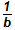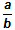# Activities

••• ##### Subject Area

• Math: Elementary Math: Fractions

• ##### AuthorK-5

• ##### Device
•TI-Nspire™ CX/CX II
•TI-Nspire™ CX CAS/CX II CAS
• ##### Software

TI-Nspire™
TI-Nspire™ CAS

## Fractions and Unit Squares

#### Activity Overview

This activity is intended to extend the concept of fraction to unit squares, where the unit fraction fraction is a portion of the area of a unit square.

A unit on a number line can be related to a unit square with sides the same length as the unit on a corresponding number line. This TI-Nspire lesson is intended to extend the concept of fraction to unit squares, where the unit fractionis a portion of the area of a unit square. In, b indicates the number of regions – which have equal area – into which the unit square has been divided, and a represents the number of those regions that are shaded.